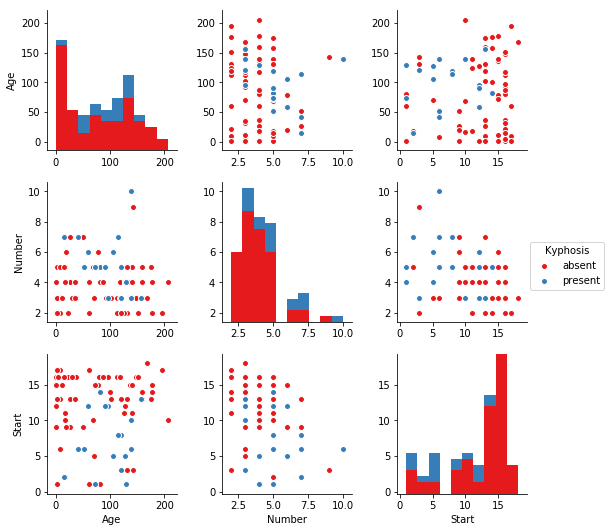# Introduction to Decision Trees and Random Forests

Wikipedia – “Decision tree learning uses a decision tree (as a predictive model) to go from observations about an item (represented in the branches) to conclusions about the item’s target value (represented in the leaves). Tree models where the target variable can take a discrete set of values are called classification trees; in these tree structures, leaves represent class labels and branches represent conjunctions of features that lead to those class labels. Decision trees where the target variable can take continuous values (typically real numbers) are called regression trees.Entropy and Information Gain are the mathematical methods of choosing the best split (For more information, please refer to http://www.saedsayad.com/decision_tree.htm. To improve performance of decision trees, we can use many trees with a random sample of features chosen as the split (Random Forests). This means that a new random sample of features is chosen for every single tree at every single split. For classification, m is typically chosen to be the square root of p. The purpose of Random Forests is that suppose there is one very strong feature in the data set. When using “bagged” trees, most of the trees will use that feature as the top split, resulting in an ensemble of similar trees that are highly correlated. Averaging highly correlated quantities does not significantly reduce variance. By randomly leaving out candidate features from each split, Random Forests “decorrelates” the trees, such that the averaging process can reduce the variance of the resulting model.

# Case Study

We’ll start by taking a look at a small sample data of Kyphosis (a medical spine condition) patients and try to predict whether or not a corrective spine surgery was successful

# 1. Preparation & Analysis

In :
```import numpy as np
import pandas as pd
import matplotlib.pyplot as plt
import seaborn as sns
%matplotlib inline
```
In :
```df = pd.read_csv('kyphosis.csv')
```
In :
```df.head()
```
Out:
Kyphosis Age Number Start
0 absent 71 3 5
1 absent 158 3 14
2 present 128 4 5
3 absent 2 5 1
4 absent 1 4 15
In :
```sns.pairplot(df,hue='Kyphosis',palette='Set1')
```
Out:
`<seaborn.axisgrid.PairGrid at 0x1a1e21eba8>`# 2. Decision Trees and Random Forest

### Splitting Data

In :
```from sklearn.model_selection import train_test_split
```
In :
```X = df.drop('Kyphosis',axis=1)
y = df['Kyphosis']
```
In :
```X_train, X_test, y_train, y_test = train_test_split(X, y, test_size = 0.3)
```

### Decision Trees

Training a single decision tree.

In :
```from sklearn.tree import DecisionTreeClassifier
```
In :
```dtree = DecisionTreeClassifier()
```
In :
```dtree.fit(X_train,y_train)
```
Out:
```DecisionTreeClassifier(class_weight=None, criterion='gini', max_depth=None,
max_features=None, max_leaf_nodes=None,
min_impurity_decrease=0.0, min_impurity_split=None,
min_samples_leaf=1, min_samples_split=2,
min_weight_fraction_leaf=0.0, presort=False, random_state=None,
splitter='best')```

### Prediction and Evaluation

In :
```pred = dtree.predict(X_test)
```
In :
```from sklearn.metrics import classification_report, confusion_matrix
```
In :
```print(classification_report(y_test,pred))
print(confusion_matrix(y_test,pred))
```
```             precision    recall  f1-score   support

absent       0.72      0.72      0.72        18
present       0.29      0.29      0.29         7

avg / total       0.60      0.60      0.60        25

[[13  5]
[ 5  2]]
```

### Tree Visualisation

Scikit learn actually has some built-in visualization capabilities for decision trees. It requires you to install the pydot library!

In :
```#from IPython.display import Image
#from sklearn.externals.six import StringIO
#from sklearn.tree import export_graphviz
#import pydot

#features = list(df.columns[1:])
#features

#dot_data = StringIO()
#export_graphviz(dtree, out_file=dot_data,feature_names=features,filled=True,rounded=True)

#graph = pydot.graph_from_dot_data(dot_data.getvalue())
#Image(graph.create_png())
```

### Random Forests

As datasets get larger and larger, random forests will almost always outperform the decision trees

In :
```from sklearn.ensemble import RandomForestClassifier
```
In :
```rfc = RandomForestClassifier(n_estimators=200)
```
In :
```rfc.fit(X_train,y_train)
```
Out:
```RandomForestClassifier(bootstrap=True, class_weight=None, criterion='gini',
max_depth=None, max_features='auto', max_leaf_nodes=None,
min_impurity_decrease=0.0, min_impurity_split=None,
min_samples_leaf=1, min_samples_split=2,
min_weight_fraction_leaf=0.0, n_estimators=200, n_jobs=1,
oob_score=False, random_state=None, verbose=0,
warm_start=False)```
In :
```rfc_pred = rfc.predict(X_test)
```
In :
```print(confusion_matrix(y_test,rfc_pred))
```
```[[16  2]
[ 5  2]]
```
In :
```print(classification_report(y_test,rfc_pred))
```
```             precision    recall  f1-score   support

absent       0.76      0.89      0.82        18
present       0.50      0.29      0.36         7

avg / total       0.69      0.72      0.69        25

```